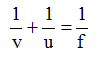# Physics

### Chapter : 3. Light Reflection

#### Numerical Method In Spherical Mirror

Numerical Method In Spherical Mirror
(A) Mirror formula
Definition : The equation relating the object distance (u) the image distance (v) and the mirror focal length (f) is called the mirror formula.(i) The mirror has a small aperture.
(ii) The object lies close to principal axis of the mirror.
(iii) The incident rays make small angles with the mirror surface or the principal axis.
(B) linear magnification For spherical mirrors
Definition : The ratio of the size of the image, as formed by reflection from the mirror to the size of the object, is called linear magnification produced by the mirror. It is represented by the symbol m.(C) Power of mirror
Power of a mirror [in Diopters] = 1/f in metre